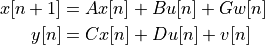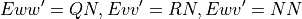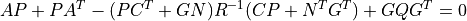# control.matlab.dlqe¶

control.matlab.dlqe(A, G, C, QN, RN[, N])[source]

Linear quadratic estimator design (Kalman filter) for discrete-time systems. Given the systemwith unbiased process noise w and measurement noise v with covariancesThe dlqe() function computes the observer gain matrix L such that the stationary (non-time-varying) Kalman filterproduces a state estimate x_e[n] that minimizes the expected squared error using the sensor measurements y. The noise cross-correlation NN is set to zero when omitted.

Parameters
• A (2D array_like) – Dynamics and noise input matrices

• G (2D array_like) – Dynamics and noise input matrices

• QN (2D array_like) – Process and sensor noise covariance matrices

• RN (2D array_like) – Process and sensor noise covariance matrices

• NN (2D array, optional) – Cross covariance matrix (not yet supported)

• method (str, optional) – Set the method used for computing the result. Current methods are ‘slycot’ and ‘scipy’. If set to None (default), try ‘slycot’ first and then ‘scipy’.

Returns

• L (2D array (or matrix)) – Kalman estimator gain

• P (2D array (or matrix)) – Solution to Riccati equation• E (1D array) – Eigenvalues of estimator poles eig(A - L C)

Notes

The return type for 2D arrays depends on the default class set for state space operations. See `use_numpy_matrix()`.

Examples

```>>> L, P, E = dlqe(A, G, C, QN, RN)
>>> L, P, E = dlqe(A, G, C, QN, RN, NN)
```